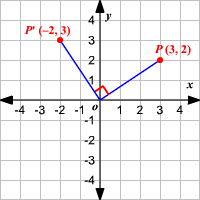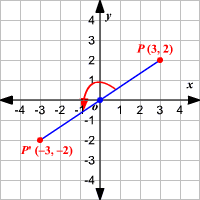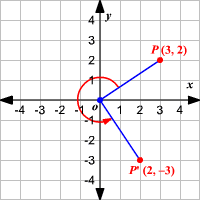# Rotations

A rotation is a transformation in a plane that turns every point of a preimage through a specified angle and direction about a fixed point. The fixed point is called the center of rotation . The amount of rotation is called the angle of rotation and it is measured in degrees. Use a protractor to measure the specified angle counterclockwise.

Some simple rotations can be performed easily in the coordinate plane using the rules below.

### Rotation by $90°$ about the origin:

A rotation by $90°$ about the origin is shown.The rule for a rotation by $90°$ about the origin is $\left(x,y\right)\to \left(-y,x\right)$ .

### Rotation by $180°$ about the origin:

A rotation by $180°$ about the origin is shown.The rule for a rotation by $180°$ about the origin is $\left(x,y\right)\to \left(-x,-y\right)$ .

### Rotation by $270°$ about the origin:

A rotation by $270°$ about the origin is shown.The rule for a rotation by $270°$ about the origin is $\left(x,y\right)\to \left(y,-x\right)$ .